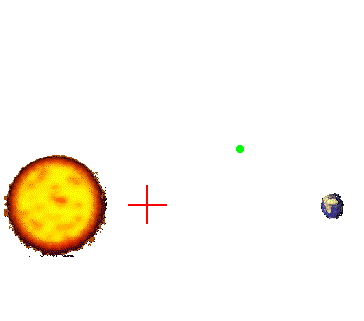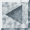Background Information

The three body problem is one of the oldest problems in dynamical systems. The problem was designed to determine the behavior of three interacting masses. In the circular restricted three body problem we consider a case where one of the bodies is so much less massive than that of the other two that the behavior of the other more massive bodies are unaffected by its gravitational potential. In addition we constrain the two primary (massive) bodies to rotate about their common center of mass in circles. This scenario is good for modeling the trajectories of spacecraft in the gravitational potential of two massive bodies.In this image we see the Sun and the Earth orbiting around their common center of mass (c.o.m.). The additional massless "test particle" is represented as the spacecraft. The circular restricted three body problem allows us to analyze the possible trajectories of the spacecraft in this system.Circular Restricted Three Body menu